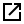# GetGeomAngleFromLength Method

## Description

Gets the tangent angle at a specified length along the geometry of the current open Item## Syntax

Visual Basic
```Public Function GetGeomAngleFromLength( _
ByVal nGeom As Integer, _
ByVal arclen As Double _
) As Double```

## Parameters

nGeom
The index of the geometry component, starting at 0.
arclen
The length along the geometry component.

## Return Type

The angle of the geometry component at the length along the geometry component, (measured in radians anti-clockwise from the current x-axis.)

## Remarks

Use GetNumGeom to get the number of geometry components in an Item.

• Items such as blocks, groups, MultiLineStrings etc can be made up of multiple geometry. Therefore the value of nGeom can be greater than 0.
• Items such as LineString items, polygons, symbols etc are made by a single piece of geometry. Therefore the value would be 0.

This method should only be used if the geometry component is two dimensional. If the geometry component is three dimensional then consider using GetGeomTgtFromLength.

Available: GEO D OD OM SISpy

Group:

## Example

sis.GetGeomAngleFromLength (0, 120)